• Python实现多条件筛选目标数据功能【测试可用】
2020-11-30 09:25:07

本文实例讲述了Python实现多条件筛选目标数据功能。分享给大家供大家参考，具体如下：

python中提供了一些数据过滤功能，可以使用内建函数，也可以使用循环语句来判断，或者使用pandas库，当然在有些情况下使用pandas是为了提高工作效率。举例如下：

a = [('chic', 'JJ'), ('although', 'IN'), ('menu', 'JJ'), ('items', 'NNS'), ('doesnt', 'JJ'),

('scream', 'NN'), ('french', 'JJ'), ('cuisine', 'NN')]

这里的a为一个list，列表中还有元组。每一个元组由单词和其词性组成，我们要筛选词性为JJ何NN的单词。可以有三种写法：

第一种，使用内建函数filter：

# -*- coding:utf-8 -*-

#!python3

a = [('chic', 'JJ'), ('although', 'IN'), ('menu', 'JJ'), ('items', 'NNS'), ('doesnt', 'JJ'),

('scream', 'NN'), ('french', 'JJ'), ('cuisine', 'NN')]

def filt_nn(data_text):

nn_data = filter(lambda x: x == 'NN'or x == 'JJ', data_text)

# print(list(nn_data))

return list(nn_data)

print(filt_nn(a))

运行结果：

[('chic', 'JJ'), ('menu', 'JJ'), ('doesnt', 'JJ'), ('scream', 'NN'), ('french', 'JJ'), ('cuisine', 'NN')]

第二种，使用pandas包：

# -*- coding:utf-8 -*-

#!python3

import pandas as pd

a = [('chic', 'JJ'), ('although', 'IN'), ('menu', 'JJ'), ('items', 'NNS'), ('doesnt', 'JJ'),

('scream', 'NN'), ('french', 'JJ'), ('cuisine', 'NN')]

data = pd.DataFrame(a, columns=['word', 'ps'])

print(data[data.ps.isin(['JJ', 'NN'])].word)

运行结果：

0 chic

4 doesnt

5 scream

6 french

7 cuisine

Name: word, dtype: object

第三种，使用循环：

# -*- coding:utf-8 -*-

#!python3

a = [('chic', 'JJ'), ('although', 'IN'), ('menu', 'JJ'), ('items', 'NNS'), ('doesnt', 'JJ'),

('scream', 'NN'), ('french', 'JJ'), ('cuisine', 'NN')]

absd = []

for i in a:

if i == 'NN' or i == 'JJ':

absd.append(i)

print(absd)

得到的结果都相同，如下：

['chic', 'menu', 'doesnt', 'scream', 'french', 'cuisine']

虽然结果相同，但是推荐第一、二种方法，因为这两个方法速度更快。

更多关于Python相关内容可查看本站专题：《Python列表(list)操作技巧总结》、《Python字符串操作技巧汇总》、《Python数据结构与算法教程》、《Python函数使用技巧总结》、《Python入门与进阶经典教程》及《Python文件与目录操作技巧汇总》

希望本文所述对大家Python程序设计有所帮助。

时间： 2018-06-10

更多相关内容
• 在日常工作中，面对Excel表格数据，为了分类进行统计，通过对表格数据筛选获取分类条目，再按条目实行汇总统计。要完成上面的工作，有人工操作和开发程序两种方法，本文通过一个实例，详细介绍这两种方法。

### Excel VBA 多条件筛选 AdvancedFilter 汇总统计 sumproduct Range与Array交换

在日常工作中，面对Excel表格数据，为了分类进行统计，通过对表格数据筛选获取分类条目，再按条目实行汇总统计。要完成上面的工作，有人工操作和开发程序两种方法，本文通过一个有10000行数据的管线调查表，对不同管径、管材进行分类统计数量和长度，详细介绍人工操作和VBA程序开发这两种方法。

# 1、人工操作方法

## 1.1高级筛选

Excel菜单：数据-高级  找开高级筛选对话框，如下图：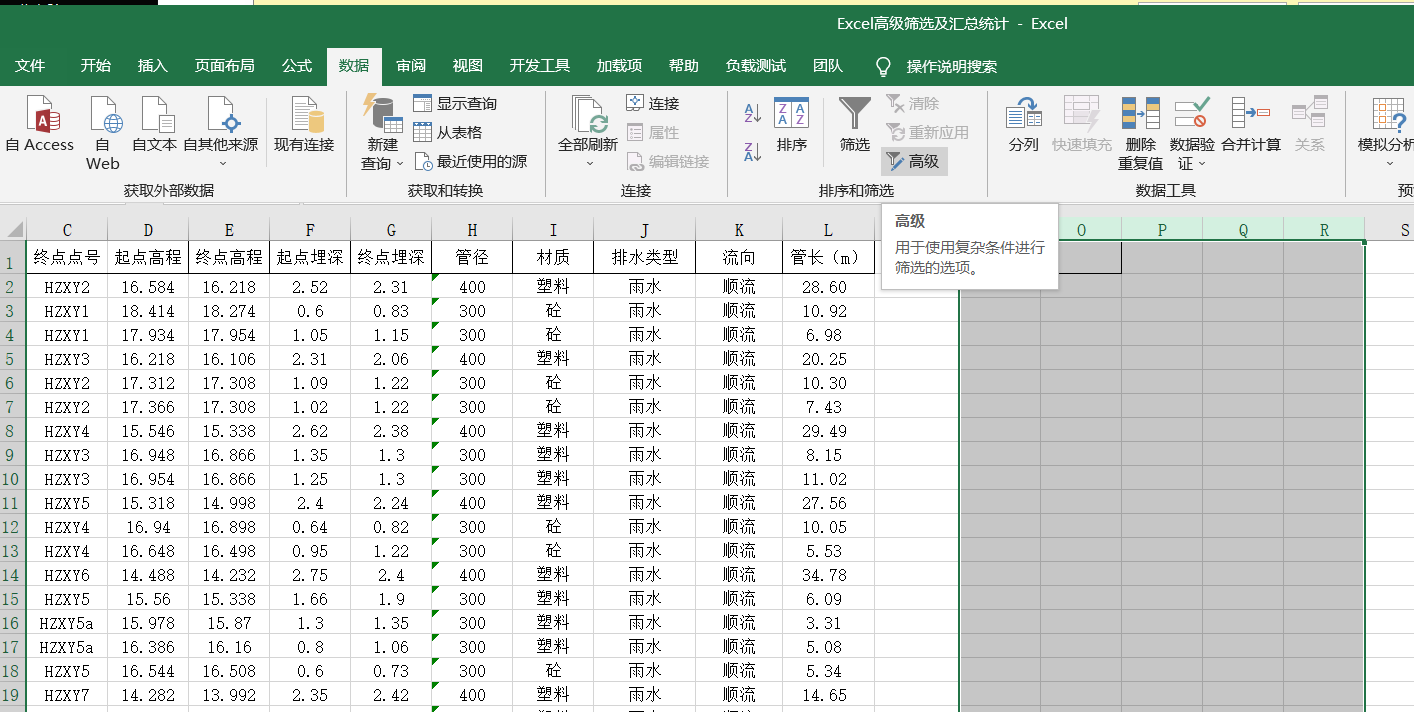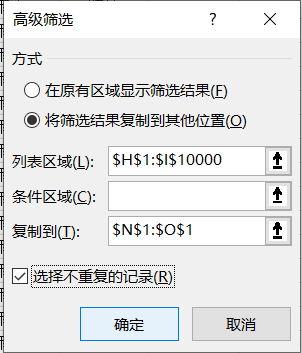对话框中：  方式：将筛选 结果复制到其他位置

列表区域： 选择需要筛选 的区域

复制到：筛选结果粘贴位置（没有数据的空白区域）

选择不重复的记录：打钩

确定后得到的结果如下图## 1.2分类统计

在P2单元格输入公式：=SUMPRODUCT((H:H=N2)*(I:I=O2),L:L)

在Q2单元格输入公式：=SUMPRODUCT((H:H=N2)*(I:I=O2))

以此类推就可以计算出所有统计数据

# 2、VBA编程的方法

虽然人工操作方法也很方便，但当我们利用程序处理一系列复杂工作的同时，要进行分类统计时，就无法使用人工操作方法了。所以还要讨论一下编程的方法。利用Excel的VBA二次开发编写程序，实现多条件筛选分类统计可以有多种方法，本文介绍宏表函数法的数组法二种方法。

## 2.1宏表函数法

宏表函数法就是人工操作法录制宏，再对宏进行修改的方法。先做统计条目的筛选，对筛选结果进行排序，最后进行统计计算。代码如下：

Sub 多条件筛选汇总统计()  '利用宏表函数进行多条件筛选汇总统计用约：最大行设10000时0.1秒；用整列计算用时1.46秒
Dim 筛选数据区域 As Range
Dim 复制区域 As Range
Dim 总长 As Double
Sheets("Sheet1").Select
sngStart = Timer
Set 筛选数据区域 = Range(Cells(1, 8), Cells(10000, 9))
Range(Cells(1, 14), Cells(30, 17)).Clear
Set 目标区域 = Range(Cells(1, 14), Cells(1, 14))
'排序
With ActiveSheet.Sort
.SortFields.Clear
.SetRange Range("N2:O10000")
.MatchCase = False
.Orientation = xlTopToBottom
.SortMethod = xlPinYin      'xlPinYin 表示按照首字母 排序   xlStroke 表示按每个字符的笔划数量排序。
.Apply
End With
i = 2
Do While Cells(i, 14) <> ""   '利用宏表函数进行统计汇总
'预先能知道最大值时，条件式及统计项均使用最大值，可提高运算速度,用时 0.1 秒
Cells(i, 16) = "=SUMPRODUCT((R2C8:R20000C8=R" + Trim(str(i)) + "C14)*(R2C9:R20000C9=R" + Trim(str(i)) + "C15),R2C12:R20000C12)"
Cells(i, 17) = "=SUMPRODUCT((R2C8:R20000C8=R" + Trim(str(i)) + "C14)*(R2C9:R20000C9=R" + Trim(str(i)) + "C15))"

'预先不知道有多少行时，条件式及统计项均使用整列，会降低运算速度,用时 1.46 秒
'Cells(i, 16) = "=SUMPRODUCT((C8:C8=R" + Trim(str(i)) + "C14)*(C9:C9=R" + Trim(str(i)) + "C15),C12:C12)"
'Cells(i, 17) = "=SUMPRODUCT((C8:C8=R" + Trim(str(i)) + "C14)*(C9:C9=R" + Trim(str(i)) + "C15))"

i = i + 1
Loop
Cells(i, 16) = WorksheetFunction.Sum(Range(Cells(2, 16), Cells(i - 1, 16)))
Cells(i, 17) = WorksheetFunction.Sum(Range(Cells(2, 17), Cells(i - 1, 17)))
Debug.Print "耗费时间: " & Timer - sngStart
Cells(1, 14) = "管径"
Cells(1, 15) = "材质"
Cells(1, 16) = "长度  m"
Cells(1, 17) = "数量"
Cells(i, 15) = "合计："
End Sub

***利用宏表函数进行条件筛选的结果只能复制到工作表的区域内，无法利用变量接收。

理解宏表函数的语法对于宏表函数中动态地址的处理很重要，现在解释一下统计宏表函数的含义：

"=SUMPRODUCT((R2C8:R20000C8=R" + Trim(str(i)) + "C14)*(R2C9:R20000C9=R" + Trim(str(i)) + "C15),R2C12:R20000C12)"

上面这句代码其实就是一段符合宏表函数语法的字符串，他等同于下面的字符串。

=SUMPRODUCT((R2C8:R20000C8=R2C14)*(R2C9:R20000C9=R2C15),R2C12:R20000C12)

红色部份是一个查询条件，意思是：第8列的第二行到20000行=第14列第2行，也应是说（管径=“400”）

绿色部份也是一个查询条件，意思是：第9列的第二行到20000行=第15列第2行，也应是说（管材=“塑料”）

黄色部份是需要统计的区域，这时是统计符合条件的管线长度。

统计数量时，不需要统计区域。

***查询条件还可以更多，每个查询条件用小括号括起来，两个条件中间用“*”相连接。

***查询条件中，把数值转化为字符串，一定要去除两端的空串，如Trim(str(i))，否则会出错。

## 2.2数组法

数组法是纯编程的方法，创建动态数组，筛选出唯一的统计条目，同时进行数据的统计，最后对结果进行排序，使统计结果按排序的要求顺序输出，本例是升序。代码如下：

Sub 综合数组分类统计()    '数组排序用时约 0.14 秒，内置函数排序用时约 0.031秒。
Dim i As Integer, j As Integer
Dim 总长 As Double, 数量 As Integer
Dim str(3)
Dim DataV(), js As Integer
On Error Resume Next
Sheets("Sheet1").Select
Dim sngStart As Single: sngStart = Timer
'筛选并排序：管径分类，材质分类
js = 0
i = 2
Do While Cells(i, 1) <> ""
For j = 1 To js
If Cells(i, 8) = DataV(0, j) And Cells(i, 9) = DataV(1, j) Then
DataV(2, j) = DataV(2, j) + Cells(i, 12)
DataV(3, j) = DataV(3, j) + 1
GoTo 20
End If
Next
js = js + 1
ReDim Preserve DataV(3, js)
DataV(0, js) = Cells(i, 8)
DataV(1, js) = Cells(i, 9)
DataV(2, js) = Cells(i, 12)
DataV(3, js) = 1
20: i = i + 1
Loop

'数组排序
Dim m1 As String, m2 As String
For i = 1 To js
For j = 1 To js - 1
m1 = DataV(0, j) + "|" + DataV(1, j)
m2 = DataV(0, j + 1) + "|" + DataV(1, j + 1)
If m1 > m2 Then
str(0) = DataV(0, j): DataV(0, j) = DataV(0, j + 1): DataV(0, j + 1) = str(0)
str(1) = DataV(1, j): DataV(1, j) = DataV(1, j + 1): DataV(1, j + 1) = str(1)
str(2) = DataV(2, j): DataV(2, j) = DataV(2, j + 1): DataV(2, j + 1) = str(2)
str(3) = DataV(3, j): DataV(3, j) = DataV(3, j + 1): DataV(3, j + 1) = str(3)
End If
Next
Next
Range(Cells(1, 14), Cells(js + 1, 17)).Value = Application.Transpose(DataV)
Cells(js + 2, 16) = WorksheetFunction.Sum(Range(Cells(2, 16), Cells(i + 1, 16)))
Cells(i + 2, 17) = WorksheetFunction.Sum(Range(Cells(2, 17), Cells(i + 1, 17)))
Debug.Print "耗费时间: " & Format(Timer - sngStart, "0.0000000000")
Cells(1, 14) = "管径"
Cells(1, 15) = "材质"
Cells(1, 16) = "长度  m"
Cells(1, 17) = "数量"
Cells(i + 2, 15) = "合计："
End Sub


展开全文数据库开发
• 总结一下Filter函数的使用。 Staff表数据如下： 1. 使用个FILTER实现嵌套筛选

总结一下Filter函数多个筛选条件的使用。

Staff表所有数据如下：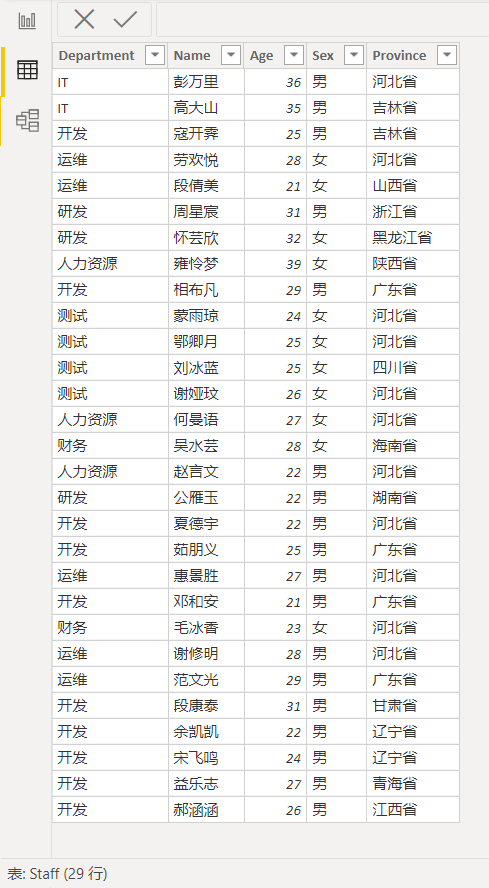1. 使用多个FILTER实现嵌套筛选

第一层筛选的是Department为“开发”的人员，第二层筛选Province是“广东省”的人员：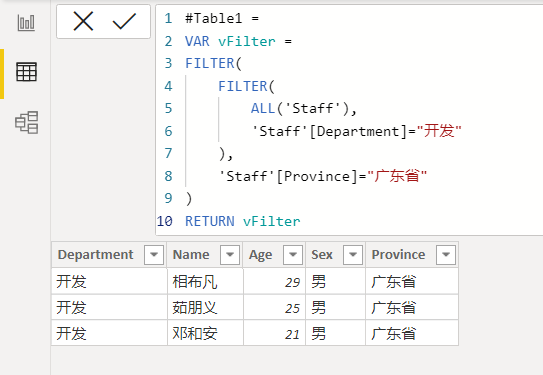2. 在FILTER中使用AND和OR实现多条件筛选

效果跟上面是一样的，但是AND函数参数只能为2个。同时满足两个条件的才会被筛选出来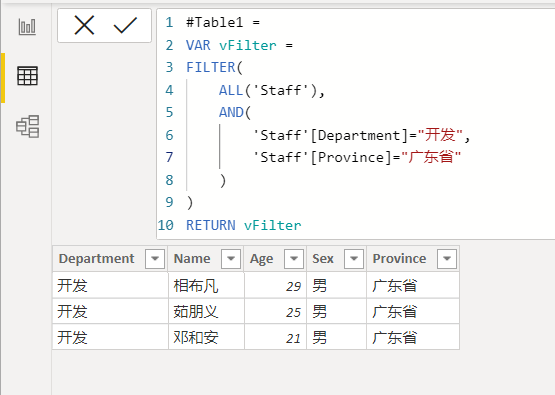使用OR，只要满足Department为“开发”或者Province为“广东省”的数据即被筛选出来，

跟AND一样，OR参数也只能为2个。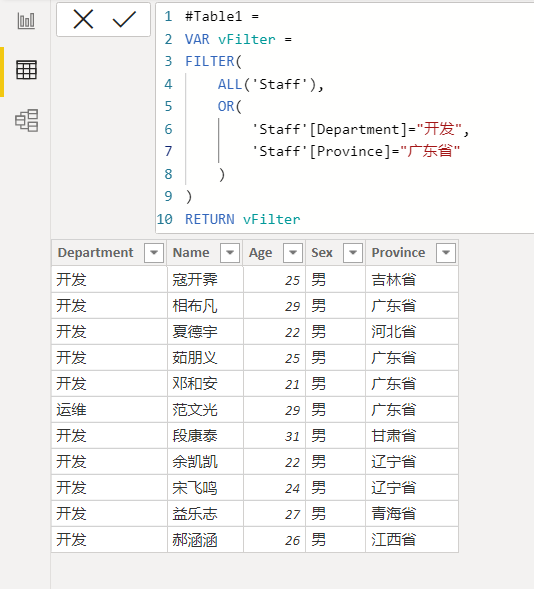3. 在FILTER中使用IN函数筛选数据

筛选出Department为开发、运维的人员。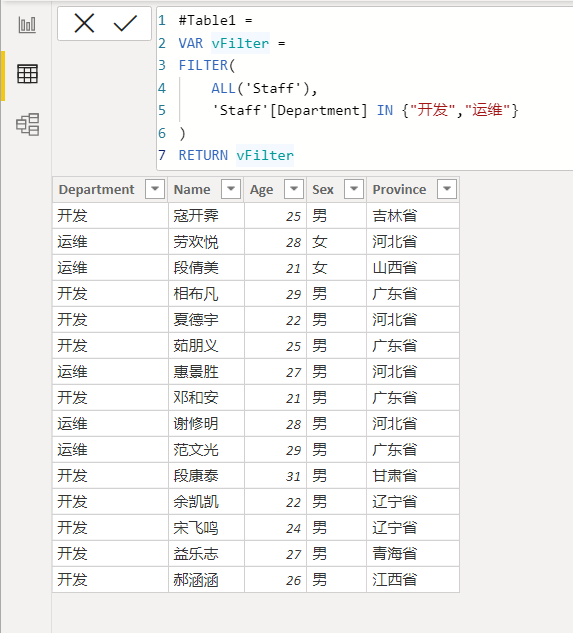组合使用：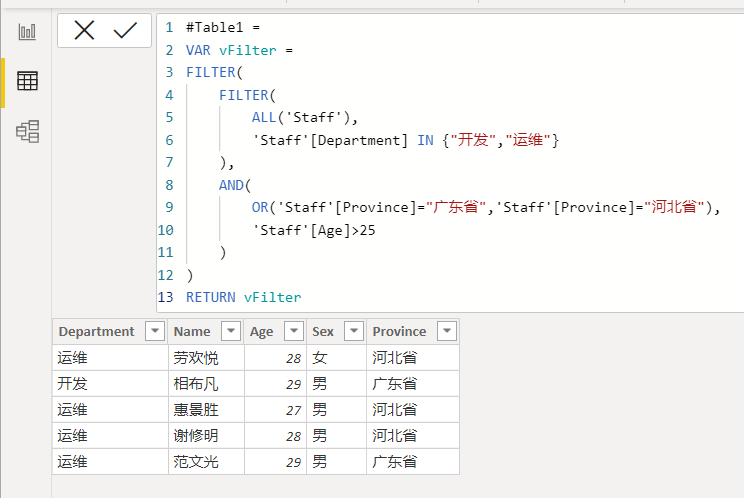2021-12-09 更新

可以直接使用"&&"串接查询条件：

FILTER(
ALL('TestTable'),
'TestTable'[Date] >= fDateFrom
&&'TestTable'[Date] <= fDateTo
&&'TestTable'[Week] = fWeek
&&'TestTable'[Min] = fMin
&&'TestTable'[Line] = fLine
&&'TestTable'[Time] = rTime
&&'TestTable'[Dir] = fDir
)

展开全文• 那么能不能实现既可以对每个成员的AP单独列表跟踪，又可以每天实时提醒重点AP及需要按时完成的AP等不同条件下的AP汇总呢？ 当然可以~~ 如果你对EXCEL VBA比较熟悉，那么可以直接看代码就可以， 如果你是新手，那就跟...
1. 经常我们会需要将一个工作簿中所有子表里满足某些条件的行或列进行筛选汇总到一个新的表格里，如果靠人力去逐条筛查，表格数据少还可以接受，但是数据表多了之后显然不太现实。这里就给大家提供一种可以进行快速汇总的方法。如果你是VBA大神，直接看代码部分就行。如果基础比较弱，可以跟着一起尝试做一遍。首先可以试着做一个样表进行试验。表格演示提供大家一个演示：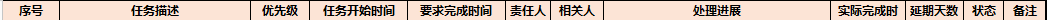2. 根据样表大家可以复制出多几个类似的样表，那么关键问题来了，如何对这些格式相同的子表进行筛选汇总呢。要解决这个问题，首先需要确定你要筛选的条件依据，本例中选择延期天数和状态进行筛选。如下
If sh.Cells(x, 10) >= -1 And sh.Cells(x, 10) <> "" And sh.Cells(x, 11) <> "关闭" Then
Set CopyRng = sh.Range(sh.Rows(x), sh.Rows(x))

3.当然我们最后想要的是将所有的子表中满足条件的行全部汇总到提醒表汇总起来。那么为了能实现遍历表格的子表，可以使用新增名称管理器的方法设定数据引用位置= GET.WORKBOOK（1），新增名称管理器的方法可以自行上网查询。获取到字表名称后就可以根据表名利用对于循环进行遍历所有表格，然后嵌套第二步的判断条件，实现对所有的表格进行筛选。为了方便，直接将所有表格按序号进行命名。

For Each sh In Sheets(Array("1.","2.","3."))
firstblankrow = sh.Range("B1").End(xlDown).Row - 1
StartRow = 2
If firstblankrow > 0 And firstblankrow > StartRow Then
For x = 2 To firstblankrow + 1
If sh.Cells(x, 10) >= -1 And sh.Cells(x, 10) <> "" And sh.Cells(x, 11) <> "关闭" Then
Set CopyRng = sh.Range(sh.Rows(x), sh.Rows(x))
1. Ok 到此就可以基本实现所有功能。附上完整代码供参考：
Private Sub Worksheet_Activate()
Dim sh As Worksheet
Dim DeSh As Worksheet
Dim x As Integer
Dim StartRow As Long
Dim Last As Long
Dim shLast As Long
Dim firstblankrow As Long
Dim CopyRng As Range
On Error Resume Next

Application.ScreenUpdating = False
Application.EnableEvents = False
Set DeSh = Me
Last = LastRow(DeSh)
If Last >= 4 Then
Rows("5:" & Last).Delete
End If
Last = 4
For Each sh In Sheets(Array("1.","2.","3."))
firstblankrow = sh.Range("B1").End(xlDown).Row - 1
StartRow = 2
If firstblankrow > 0 And firstblankrow > StartRow Then
For x = 2 To firstblankrow + 1
If sh.Cells(x, 10) >= -1 And sh.Cells(x, 10) <> "" And sh.Cells(x, 11) <> "关闭" Then
Set CopyRng = sh.Range(sh.Rows(x), sh.Rows(x))

CopyRng.Copy
With DeSh.Cells(Last + 1, "A")
.PasteSpecial xlPasteValues
.PasteSpecial xlPasteFormats
Application.CutCopyMode = xlCopy
DeSh.Cells(Last + 1, LastColumn(DeSh)).Select
End With
Last = LastRow（DeSh）
End If If
Next
End If
Next
Application.ScreenUpdating = True
Application.EnableEvents = True

ExitSub:
Application.Goto DeSh.Cells(1)
DestSh.Columns.AutoFit
Application.ScreenUpdating = True
Application.EnableEvents = True
End Sub

另外模块中还用到了两个自定义函数，分别用来定位最后一行和最后一列的序号。代码如下：

Function LastRow(sh As Worksheet)
On Error Resume Next

LastRow = sh.Cells.Find(what:="*", after:=sh.Range("A1"), lookat:=xlPart, LookIn:=xlFormulas, searchorder:=xlByRows, searchdirection:=xlPrevious, MatchCase:=False).Row

End Function

Function LastColumn(sh As Worksheet)
On Error Resume Next

LastColumn = sh.Cells.Find(what:="*", after:=sh.Range("A1"), lookat:=xlPart, LookIn:=xlFormulas, searchorder:=xlByColumns, searchdirection:=xlPrevious, MatchCase:=False).Column

End Function
1. 到这里所有的功能就可以实现了，第一次写，不对之处还请包涵。大家可以尝试一下，如果有疑问可以在评论区留言交流，上班族回复不及时还请见谅，谢谢~~
展开全文EXCEL VBA
• 实现用公式多条件筛选数据，比如有很多个工作簿，每个工作簿都有以下数据。   A B C D 1 0 1 2 44 2 1 2 3 55 3 2 3 4 66 找出A列为0，B列为1，C列为2的那一行，也就是第一行，输出D列数据44。 最后...excel 公式
• 文章介绍sumifs 函数基本用法，然后以一个综合的实例来剖析sumifs函数的详细深入使用。excel
• excel sumif函数多条件求和 sumif的高级用法：跨多表条件求和，这个问题是我们很多人都很困惑的一个问题，excel sumif函数多条件求和是我们非常常用的一个东西，也是我们工作和学习中必不可少的一个数据公式，那么...
• EXCEL如何实现同条件多列求和就按正常方法求和就行了，不用管文字，系统能识别，我经常这样求和。excel中sumifs公式列、或者区域求和1、首先在格中输入：=sumifs——回车——点击上面的【插入】标志：fx。2、求和...
• excel中截取分类汇总 在Excel中汇总过滤列表 (Total a Filtered List in Excel) In Excel, you might have a long list of orders with a grand total at the end. If you filter the Region column, so the list ...列表 python 大数据 java 机器学习
• 前面已经介绍了Excel在工作中常用的统计函数，但是在众多的统计函数中，有一种函数集合了上述常用的统计函数，它就是SUBTOTAL函数，该函数是功能强大的分类汇总统计函数。 SUBTOTAL函数 SUBTOTAL函数的语法格式为...excel 数据
• 排序与筛选一、排序二、筛选三、分类汇总 一、排序 1.按单个条件排序 操作： 选择待排序列的任意单元格，单击“开始”选型卡的“排序和筛选”按钮，选择需要的排序方式 或者在“数据”选项卡中进行类似操作。 注意：...Excel
• ## MYSQL——函数汇总

多人点赞 2022-04-25 09:29:39
文章目录 系列文章目录 前言 一、pandas是什么？ 二、使用步骤 1.... 2....博主最近在使用MySQL时...MySQL提供的内置函数从 实现的功能角度 可以分为数值函数、字符串函数、日期和时间函数、流程控制函数、加密与解密...sql 数据库 mysql
• 在A2单元格输入公式：=SUBTOTAL(3,B$1:B2)-1 再双击填充公式即可这样设置的序号，不管如何筛选，序号都是从1开始递增，效果如下：2、筛选后的加法计算——求和上图表格中普通求和我们可以利用SUM函数或者快捷键都... • 这个例子是对课程进度表里面的某个学生的剩余课时进行求和汇总。 laravel 版本是 lts 5.5 StudentLessonProgress::where('student_info_id',$student_info_id) ->sum('total_left_class_num'); 打印sql语句如下：...
• Python 中使用 loc 函数配合筛选条件来完成筛选功能。配合 sum 和 count 函数还能实现 excel 中 sumif 和 countif 函数的功能。 1）使用“与”条件进行筛选 条件是年龄大于 25 岁，并且城市为 bepython
• 筛选和排序是Excel中使用频率最多的功能，通过这个功能可以很方便的对数据表中的数据使用指定的条件进行筛选和计算，以获得需要的结果。在Pandas中通过.sort和.loc函数也可以实现这两 个功能。.sort函数可以实现对...
• 来源：吊车尾学院我整理了100个Pandas常用的函数，分别分为6类：统计汇总函数、数据清洗函数、数据筛选、绘图与元素级运算函数、时间序列函数和其他函数。统计汇总函数函数含义min()计算...python html 数据挖掘 opengl
• 主要介绍了C# DataTable常见用法，帮助大家更好的理解和学习c#，感兴趣的朋友可以了解下
• filter 用法，筛选出列表中满足条件的值, 使用list()方法将filter()筛选的结果返回 例如, 使用filter筛选出(-5,5)之间的所有条件满足大于0的数 res=list(filter(lambda x:x>0,range(-5,5))) # filter的使用 # 1....reduce
• 在Excel中我们可以很方便的对数据进行排序、筛选、分类汇总等基本操作，R语言中没有这种傻瓜式的一键操作，如何才能完成这种操作？一、排序1、单变量序列排序　单变量序列的排序常用到rank、sort和order函数。　给一...排序 dplyr
• ## EXCEL常用函数汇总

千次阅读 2020-11-17 13:24:59
EXCEL常用函数汇总（以Excel 2016版举例） 根据自己的习惯分为七大类： EXCEL常用函数 第一类：文本处理函数 Trim函数： 作用：除了单词之间的单个空格之外，移除文本中的所有空格。excel
• 这里对数据分析中常用的excel函数进行分类汇总，共五类：关联匹配类、清洗处理类、逻辑运算类、计算统计类、时间序列类 一、关联匹配类 数据不在同一个excel表或同一个excel表不同sheet中，数据太，copy麻烦也不...
• 通过在查询编辑输入”=#shared”调出帮助，然后提取列，筛选出所有的Table函数 二、Table函数分类 Table类函数：总共102个，可查基本信息查看、表操作、列操作、行操作、排序、表构建、表转换、包含关系、其他...
• 一个具有11项汇总方式的函数SUBTOTAL=SUBTOTAL(9,$B$2:B2)在数据筛选求和上有意想不到的功能，11项功能为：1、求平均数，2、求计数，3、求计数值(自动筛选序列)4、求最大值，5、求最小值，6、求乘积，7、求总体标准...
• 来源：吊车尾学院编辑：杰哥的IT之旅我整理了100个Pandas常用的函数，分别分为6类：统计汇总函数、数据清洗函数、数据筛选、绘图与元素级运算函数、时间序列函数和其他函数。统计汇总函数...
• 筛选的字段很，而且不一定筛选哪一个字段，开始就是各种if各种循环，自己看的都觉得low 于是换了一种方法，用js数组的filter函数，这里就不讲filter怎么回事了， 这里的if条件就是每种字段选中的长度，...Filter Lambda
• 当发现可以进行筛选时，语句如下： SELECT count(*) filter (where PERIOD = '01') AS business_daily, -- 日累计 业务办理 count(*) filter (where PERIOD = '02') AS business_per_month -- 月累计 业务办理 ...mysql 数据库 oracle postgresql
• 单位A 代码B 面积（㎡）C A组 011 124 A组 123 15 A组 011 356 A组 123 44 ...按照单位和代码求面积的和，可以用EXCEL中的SUM函数， ...1、可以在函数栏中先输入=SUM...
• ## sklearn函数汇总

千次阅读 2020-02-19 23:00:02
Python笔记--sklearn函数汇总Summer Memories​关注他28 人赞同了该文章1. 拆分数据集为训练集和测试集：from sklearn.model_selection import train_test_split x_train, x_test, y_train, y_test = train_test_...
• 函数及其他： 定时器 setTimeout(函数，N毫秒) 一次性的定时器，N毫秒后执行一次函数，返回定时器标识 setInterval(函数，N毫秒) 重复定时器，每N毫秒都执行一次函数，返回定时器标识 clearTimeout(定时器标识) 清除...javascript html vue.js...